# Overview

The ordered beta regression model is designed explicitly for data with upper and lower bounds, such as survey slider scales, dose/response relationships, and indexes. This type of data cannot be fit with the standard beta regression model because the beta distribution does not allow for any observations at the bounds, such as the upper limit of a scale. The ordered beta regression model solves this problem by combining the beta distribution with an ordinal distribution over continuous and discrete, or degenerate, observations at the bounds. It is an efficient model that produces intelligible estimates that also respect the bounds of the dependent variable. For more information, I refer you to my paper on the model: https://osf.io/preprints/socarxiv/2sx6y/.

This notebook contains instructions for running the ordered beta regression model in the R package ordbetareg. ordbetareg is a front-end to brms, a very powerful regression modeling package based on the Stan Hamiltonian Markov Chain Monte Carlo sampler. I only show in this vignette a small part of the features which are available via brms, and I refer the user to the copious documentation describing the features of the package. Suffice it to say that most kinds of regression models can be fit with the software, including hierarchical, dynamic, nonlinear and multivariate models (or all of the above in combination). The ordbetareg package allows for all of these features to be used with the ordered regression model distribution by adding this distribution to brms.

If you use the model in a paper, please cite it as:

Kubinec, Robert. “Ordered Beta Regression: A Parsimonious, Well-Fitting Model for Continuous Data with Lower and Upper Bounds.” Political Analysis. 2022. Forthcoming.


# whether to run models from scratch
run_model <- F

# Define Custom Family

If you prefer to run brms directly rather than use this package, you can run the underlying code via the R script define_ord_betareg.R in the paper Github repo. Please not that I cannot offer support for this alternative, although it should work.

# Data Preparation

First, I load data from a Pew Forum survey that asked a question about respondents’ views towards college professors.


data("pew")

pew %>%
ggplot(aes(x=as.numeric(therm))) +
geom_histogram(bins=100) +
theme_minimal() +
theme(panel.grid=element_blank()) +
scale_x_continuous(breaks=c(0,25,50,75,100),
labels=c("0","Colder","50","Warmer","100")) +
ylab("") +
xlab("") +
labs(caption=paste0("Figure shows the distribution of ",sum(!is.na(pew$therm))," non-missing survey responses."))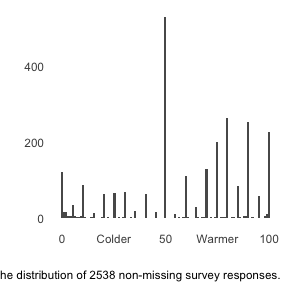The distributions of feelings towards college professors contains both degenerate (0 and 100) and continuous responses between 0 and 100. To model it, the outcome needs to be re-scaled to lie strictly between 0 and 1. However, it is not necessary to do that ahead of time as the ordbetareg package will do that re-normalization internally. I also do some other data processing tasks:  model_data <- select(pew,therm,age="F_AGECAT_FINAL", sex="F_SEX_FINAL", income="F_INCOME_FINAL", ideology="F_IDEO_FINAL", race="F_RACETHN_RECRUITMENT", education="F_EDUCCAT2_FINAL", region="F_CREGION_FINAL", approval="POL1DT_W28", born_again="F_BORN_FINAL", relig="F_RELIG_FINAL", news="NEWS_PLATFORMA_W28") %>% mutate_at(c("race","ideology","income","approval","sex","education","born_again","relig"), function(c) { factor(c, exclude=levels(c)[length(levels(c))]) }) %>% # need to make these ordered factors for BRMS mutate(education=ordered(education), income=ordered(income)) The completed dataset has 2538 observations. # Run In ordbetareg The ordbetareg function will take care of normalizing the outcome and adding additional information necessary to estimate the distribution. Any additional arguments can be passed to the underlying brm function to use specific brm features. For example, in the code below I use the backend="cmdstanr" argument to brm(), which allows me to use the R package cmdstanr for estimating models. cmdstanr tends to have the most up to date version of Stan, though you must install it yourself. What you need to pass to the ordbetareg function are the standard components of any R model: a formula and a dataset that has all the variables mentioned in the formula. There are additional parameters that allow you to modify the priors, such as for the dispersion parameter phi. While in most cases these priors are sensible defaults, if you have data with an unusual scale (such as very large or very small values), you may want to change the priors to ensure they are not having an outsize effect on your estimates. If you want to use some of brms more powerful techniques, such as multivariate modeling, you can also pass the result of a bf function call to the formula argument. I refer you to the brmsformula() function help for more details. To demonstrate some of the power of using brms as a regression engine, I will model education and income as ordinal predictors by using the mo() function in the formula definition. By doing so, we can get a single effect for education and income instead of having to use dummies for separate education/income categories. As a result, I can include an interaction between the two variables to see if wealthier more educated people have better views towards college professors than poorer better educated people. Finally, I include varying (random) census region intercepts.  if(run_model) { ord_fit_mean <- ordbetareg(formula=therm ~ mo(education)*mo(income) + (1|region), data=model_data, cores=2,chains=2,iter=1000, refresh=0) # NOTE: to do parallel processing within chains # add the options below #threads=threading(5), #backend="cmdstanr" #where threads is the number of cores per chain # you must have cmdstanr set up to do so # see https://mc-stan.org/cmdstanr/ } else { data("ord_fit_mean") } The one divergent transition referenced above is due to the well-known funnel problem of the variance of the random intercepts, and I will ignore it for the purposes of this vignette. # Post-Estimation The first thing we can do is extract the model cutpoints and overlay them on the empirical distribution to see how the model is dividing the outcome into discrete-ish categories. We have to do transformation of the cutpoints using the inverse logit function in R (plogis) to get back values in the scale of the response, and I have to exponentiate and add the first cutpoint to get the correct value for the second cutpoint:  all_draws <- prepare_predictions(ord_fit_mean) cutzero <- plogis(all_draws$dpars$cutzero) cutone <- plogis(all_draws$dpars$cutzero + exp(all_draws$dpars$cutone)) pew %>% ggplot(aes(x=therm)) + geom_histogram(bins=100) + theme_minimal() + theme(panel.grid=element_blank()) + scale_x_continuous(breaks=c(0,25,50,75,100), labels=c("0","Colder","50","Warmer","100")) + geom_vline(xintercept = mean(cutzero)*100,linetype=2) + geom_vline(xintercept = mean(cutone)*100,linetype=2) + ylab("") + xlab("") + labs(caption=paste0("Figure shows the distribution of ",sum(!is.na(pew$therm))," non-missing survey responses."))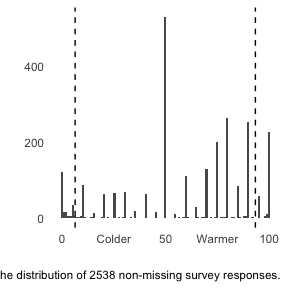We can see in the plot above that the model does a good job isolating values that are very close to the degenerate bounds of 0 and 100 from values that are more continuous in nature. Note that this plot is somewhat of a heuristic as the cutpoints are technically in a latent continuous space, but it is nonetheless helpful for seeing how spread out the data are according to the model’s estimates.

We can plot the full predictive distribution relative to the original outcome. The model can’t capture all of the modality in the distribution – there are effectively four separate modes – but it is reasonably accurate over the middle responses and the responses near the bounds.


pp_check(ord_fit_mean) + theme_minimal()
#> Using 10 posterior draws for ppc type 'dens_overlay' by default.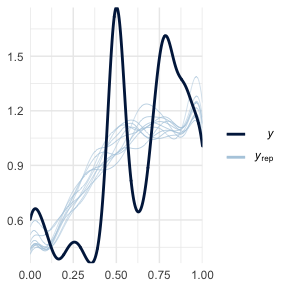We can see the coefficients from the model in table formusing the modelsummary package, which has reasonable (though not perfect) support for brms models. We’ll specify to include only confidence intervals as other frequentist statistics have no Bayesian analogue (i.e. p-values). We’ll also specify only the main effects of the ordinal predictors, and give them more informative names.


library(modelsummary)
#>
#> Attaching package: 'modelsummary'
#> The following object is masked from 'package:Hmisc':
#>
#>     Mean

modelsummary(ord_fit_mean,statistic = "conf.int",
metrics = "RMSE",
coef_map=c("bsp_moeducation"="Education",
"bsp_moincome"="Income",
"bsp_moeducation:moincome"="EducationXIncome"))
Model 1
Education 0.115
[0.035, 0.190]
Income −0.051
[−0.092, −0.013]
EducationXIncome 0.007
[−0.007, 0.022]
Num.Obs. 2431
RMSE 0.49

modelsummary tables have many more options, including output to both html and latex formats.

# Marginal Effects

There is a related package, marginaleffects, that allows us to convert these coefficients into more meaningful marginal effect estimates, i.e., the effect of the predictors expresses as the actual change in the outcome on the 0 - 1 scale. We can’t calculate marginal effects for all of the predictors as some of them are cutpoints, etc. (the function will return an error about the intercept), so we’ll focus on one of the main effects we are interested in as in the table above:


library(marginaleffects)

marg_effs <- marginaleffects(ord_fit_mean,
variables="education")

summary(marg_effs) %>% knitr::kable()
type term contrast estimate conf.low conf.high
response education Associate’s degree - Less than high school 0.0685996 0.0055266 0.1244853
response education College graduate/some postgrad - Less than high school 0.1293433 0.0706173 0.1876937
response education High school graduate - Less than high school 0.0152928 -0.0128111 0.0577985
response education Postgraduate - Less than high school 0.1990979 0.1325604 0.2623659
response education Some college, no degree - Less than high school 0.0457593 -0.0025861 0.0978661

We can see that we have separate marginal effects for each level of education due to modeling it as an ordinal predictor. At present the marginaleffects cannot calculate a single effect, though that is possible manually. As we can see with the raw coefficient in the table above, the marginal effects are all positive, though the magnitude varies across different levels of education.

# Understanding Clustering/Polarization of Respondents

As I explain in the paper, one of the main advantages of using a Beta regression model is its ability to model the dispersion among respondents not just in terms of variance (i.e. heteroskedasticity) but also the shape of dispersion, whether it is U or inverted-U shaped. Conceptually, a U shape would imply that respondents are bipolar, moving towards the extremes. An inverted-U shape would imply that respondents tend to cluster around a central value. We can predict these responses conditionally in the sample by adding predictors for phi, the scale/dispersion parameter in the Beta distribution. Higher values of phi imply a uni-modal distribution clustered around a central value, with increasing phi implying more clustering. Lower values of phi imply a bi-modal distribution with values at the extremes. Notably, these effects are calculated independently of the expected value, or mean, of the distribution, so values of phi will produce different shapes depending on the average value.

The one change we need to make to fit this model is to add a formula predicting phi in the code below. Because we now have two formulas–one for the mean and one for dispersion–I use the bf function to indicate these two sub-models. I also need to specify phi_reg to be TRUE because some of the priors will change.

Because there is no need to model the mean, I leave the first formula as therm ~ 0. I don’t specify 1 for an intercept because the ordered regression model has other intercepts (i.e., the cutpoints). I then specify a separate model for phi with an interaction between age and sex to see if these covariates are associated with dispersion.


if(run_model) {

ord_fit_phi <- ordbetareg(bf(therm ~ 0,
phi ~ age + sex),
phi_reg = T,
data=model_data,
cores=2,chains=2,iter=1000,
refresh=0)
# NOTE: to do parallel processing within chains
# add the options below
#backend="cmdstanr"
#where threads is the number of cores per chain
# you must have cmdstanr set up to do so
# see https://mc-stan.org/cmdstanr/

} else {

data("ord_fit_phi")

}

We can quickly examine the raw coefficients:


summary(ord_fit_phi)
#>  Family: ord_beta_reg
#>   Links: mu = logit; phi = log; cutzero = identity; cutone = identity
#> Formula: therm ~ 0 + Intercept + +0
#>          phi ~ age + sex
#>    Data: data (Number of observations: 2535)
#>   Draws: 2 chains, each with iter = 1000; warmup = 500; thin = 1;
#>          total post-warmup draws = 1000
#>
#> Population-Level Effects:
#>               Estimate Est.Error l-95% CI u-95% CI Rhat Bulk_ESS Tail_ESS
#> phi_Intercept     1.00      0.08     0.84     1.15 1.00      740      708
#> Intercept         0.31      0.02     0.27     0.36 1.01     1089      667
#> phi_age30M49      0.04      0.09    -0.13     0.22 1.00      778      679
#> phi_age50M64     -0.05      0.09    -0.23     0.12 1.00      736      795
#> phi_age65P       -0.14      0.09    -0.32     0.04 1.00      793      652
#> phi_sexFemale     0.13      0.05     0.02     0.23 1.00     1012      802
#>
#> Family Specific Parameters:
#>         Estimate Est.Error l-95% CI u-95% CI Rhat Bulk_ESS Tail_ESS
#> cutzero    -2.69      0.10    -2.89    -2.50 1.00      648      573
#> cutone      1.67      0.02     1.63     1.71 1.00      770      768
#>
#> Draws were sampled using sampling(NUTS). For each parameter, Bulk_ESS
#> and Tail_ESS are effective sample size measures, and Rhat is the potential
#> scale reduction factor on split chains (at convergence, Rhat = 1).

However, these are difficult to interpret as they relate to the Beta distribution, which is highly nonlinear. Generally speaking, higher values of phi mean the distribution is more concentrated around a single point. Lower values imply the distribution is more dispersed to the point that it actually becomes bi-modal, meaning that responses could be close to either 0 or 1 but are unlikely in the middle.

Because phi is a dispersion parameter, by definition the covariates have no effect on the average value. As a result, we’ll need to use the posterior_predict function in brms if we want to get an idea what the covariates do. We don’t have any fancy packages to do this for us, so we’ll have to pass in two data frames, one with sex equal to female and one with sex equal to male. We’ll want each data frame to have each unique value of age in the data.


# we can use some dplyr functions to make this really easy

female_data <- distinct(model_data, age) %>%
mutate(sex="Female")

male_data <- distinct(model_data, age) %>%
mutate(sex="Male")

to_predict <- bind_rows(female_data,
male_data) %>%
filter(!is.na(age))

pred_post <- posterior_predict(ord_fit_phi,
newdata=to_predict)

# better with iterations as rows

pred_post <- t(pred_post)
colnames(pred_post) <- 1:1000

# need to convert to a data frame

data_pred <- as_tibble(pred_post) %>%
mutate(sex=to_predict$sex, age=to_predict$age) %>%
gather(key="iter",value='estimate',-sex,-age)

data_pred %>%
ggplot(aes(x=estimate)) +
geom_density(alpha=0.5,aes(fill=sex)) +
theme(panel.background = element_blank(),
panel.grid=element_blank())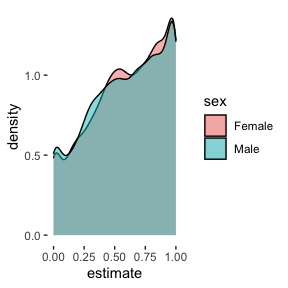We can see that the female distribution is more clustered around a central value – 0.5 – than are men, who are somewhat more likely to be near the extremes of the data. However, the movement is modest, as the value of the coefficient suggests. Regression on phi is useful when examining polarizing versus clustering dynamics in the data.

# Simulate Data

Finally, we can also simulate data from the ordered beta regression model with the sim_ordbeta function. This is useful either for examining how different parameters interact with each other, or more generally for power calculation by iterating over different possible sample sizes. I demonstrate the function here, though note that the vignette loads saved simulation results unless run_model is set to TRUE. Because each simulation draw has to estimate a model, it can take some time to do calculations. Using multiple cores a la the cores option is strongly encouraged to reduce processing time.

To access the data simulated for each run, the return_data=TRUE option can be set. To get a single simulated dataset, simply use this option combined with a single iteration and set of parameter values. The data are saved as a list in the column data in the returned data frame. The chunk below examines the first 10 rows of a single simulated dataset (note that the rows are repeated k times for each iteration, while there is one unique simulated dataset per iteration). Each predictor is listed as a Var column from 1 to k, while the simulated outcome is in the outcome column.


# NOT RUN IN THE VIGNETTE

single_data <- sim_ordbeta(N=100,iter=1,
return_data=T)

# examine the first dataset

knitr::kable(head(single_data\$data[]))

By default, the function simulates continuous predictors. To simulate binary variables, such as in a standard experimental design, use the beta_type function to specify "binary" predictors and treat_assign to determine the proportion assigned to treatment for each predictor. For a standard design with only one treatment variable, we’ll also specify that k=1 for a single covariate. Finally, to estimate a reasonable treatment effect, we will specify that beta_coef=0.5, which equals an increase of .5 on the logit scale. While it can be tricky to know a priori what the marginal effect will be (i.e., the actual change in the outcome), the function will calculate marginal effects and report them, so you can play with other options to see what gets you marginal effects/treatment effects of interest.


if(run_model) {

sim_data <- sim_ordbeta(N=c(250,500,750),
k=1,
beta_coef = .5,
iter=100,cores=10,
beta_type="binary",
treat_assign=0.3)

} else {

data("sim_data")

}

For example, in the simulation above, the returned data frame stores the true marginal effect in the marg_eff column, and lists it as 0.284, which is quite a large effect for a $$[0,1]$$ outcome. The following plot shows some of the summary statistics derived by aggregating over the iterations of the simulation. Some of the notable statistics that are included are power (for all covariates $$k$$), S errors (wrong sign of the estimated effect) and M errors (magnitude of bias). As can be seen, issues of bias decline markedly for this treatment effect size and a sample of 500 or greater has more than enough power.


sim_data %>%
select(Proportion S Errors="s_err",N,Power="power",
M Errors="m_err",Variance="var_marg") %>%
gather(key = "type",value="estimate",-N) %>%
ggplot(aes(y=estimate,x=N)) +
#geom_point(aes(colour=model),alpha=0.1) +
stat_summary(fun.data="mean_cl_boot") +
ylab("") +
xlab("N") +
scale_x_continuous(breaks=c(250,500,750)) +
scale_color_viridis_d() +
facet_wrap(~type,scales="free_y",ncol = 2) +
labs(caption=stringr::str_wrap("Summary statistics calculated as mean with bootstrapped confidence interval from simulation draws. M Errors  and S errors are magnitude of bias (+1 equals no bias) and incorrect sign of the estimated marginal effect respectively. Variance refers to estimated posterior variance (uncertainty) of the marginal effect(s).",width=50)) +
guides(color=guide_legend(title=""),
linetype=guide_legend(title="")) +
theme_minimal() +
theme(plot.caption = element_text(size=7),
axis.text.x=element_text(size=8))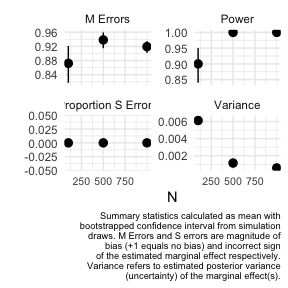While the sim_ordbeta function has the ability to iterate over N, it is of course possible to do more complex experiments by wrapping the function in a loop and passing different values of other parameters, such as treat_assign.